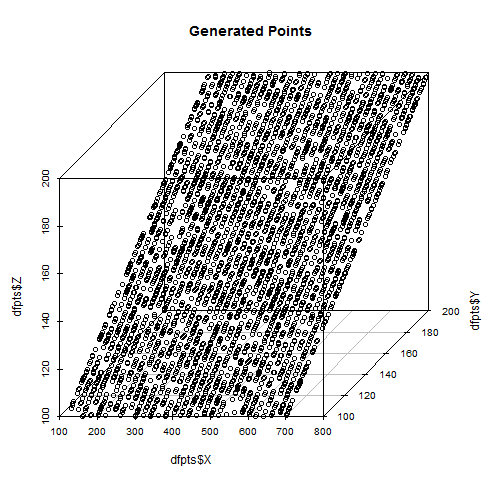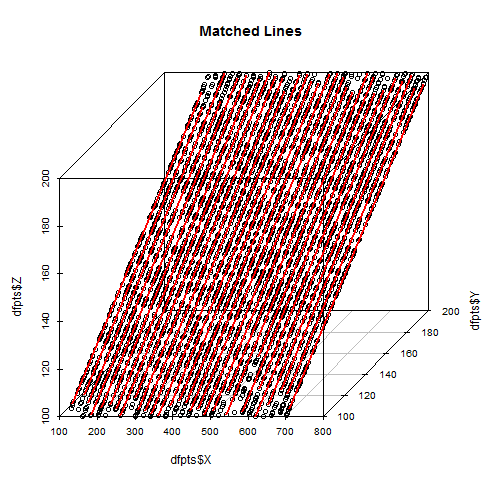# Linear Clustering

Jaromir D.B. Nemec

Started on 2016-07-17 10:22:48.

Given data points in a three dimensional plane define a k lines so that a maximum number of points are covered with the lines. A points is covered with a line if the distance of the points from the line is below a defined limit.

We will use following parameters to define the line:

Parameters:

line width - line_width/2 is the allowed distance of the point from the ideal line = 0.6

minimum line length - points with shorter distance are not considered to belong to the same line = 140

maximum line length - points with longer distance are not considered to belong to the same line = 175

minimum points on a line - lines with less points are ignored = 18

### Sample Data

The sample data is stored in the csv file linear_clustering_3d.csv with the following layout:

X,Y,Z coordinates of the point

``````##        X                Y                Z
##  Min.   :122.49   Min.   :100.03   Min.   :100.03
##  1st Qu.:309.56   1st Qu.:126.18   1st Qu.:126.18
##  Median :458.91   Median :150.96   Median :150.96
##  Mean   :460.75   Mean   :150.75   Mean   :150.75
##  3rd Qu.:608.90   3rd Qu.:174.98   3rd Qu.:174.98
##  Max.   :798.70   Max.   :199.98   Max.   :199.98
``````
``````## 'data.frame':    4500 obs. of  3 variables:
##  \$ X: num  217 136 199 215 170 ...
##  \$ Y: num  197 116 179 195 150 ...
##  \$ Z: num  197 116 179 195 150 ...
``````

To speed up the processing we will use only 20% sample of the data.

``````##        X                Y                Z
##  Min.   :123.77   Min.   :100.16   Min.   :100.16
##  1st Qu.:305.48   1st Qu.:125.47   1st Qu.:125.47
##  Median :455.08   Median :152.16   Median :152.16
##  Mean   :458.23   Mean   :151.12   Mean   :151.12
##  3rd Qu.:612.73   3rd Qu.:175.49   3rd Qu.:175.49
##  Max.   :796.58   Max.   :199.98   Max.   :199.98
``````
``````## 'data.frame':    900 obs. of  3 variables:
##  \$ X: num  653 405 323 504 579 ...
##  \$ Y: num  153 105 103 104 199 ...
##  \$ Z: num  153 105 103 104 199 ...
``````

## Graph Data

The complete set of the data points is shown below in 3D.## Approach

I developed the following algorithm using a dedicated distance measure.

Following steps are performed:

1) Define a distance metric returning:

zero - if the points do not belong to a line

Euclidian distance of the points - if the points constitute a line according to the defined parameters, i.e.

``````- their distance is higher or equal than the *min_line_length* and

- their distance is lower or equal than the *max_line_length* and

- the line consists or at least *min_line_points* points with a distance lower that *line_width/2* from the line
``````

2) Calculate distance matrix using this distance measure (use sample of the data for large data sets; adjust the line parameters accordingly)

3) Find the points A and B with maximum distance - break to step 5) if the distance is zero

Note that if the distance is higher than zero the points A and B are building a line based on our definition

4) Get all points belonging to the line AB and remove them from the distance matrix. Repeat the step 3) to find another line

5) Check the coverage of the point with the selected lines, if substantial number of points remains uncovered, repeat the whole algorithm with adjusted line parameters.

6) In case that data sample was used - reassign all points to the lines and recalculate the boundary points.

## Define Distance Measure

Step 1 - Here we define the distance measure

``````## distance of point p from line (a,b) in 3D
## see
## http://stackoverflow.com/questions/35194048/using-r-how-to-calculate-the-distance-from-one-point-to-a-line

dist3d <- function(p,a,b) {
##print(paste ("in dist3d p ",  (p), "a ",  (a), "b ",  (b)))
v1 <- a - b
v2 <- p - a
v3 <- cross3d_prod(v1,v2)
area <- sqrt(sum(v3*v3))/2
d <- 2*area/sqrt(sum(v1*v1))
}

cross3d_prod <- function(v1,v2){
v3 <- vector()
v3 <- v1*v2-v1*v2
v3 <- v1*v2-v1*v2
v3 <- v1*v2-v1*v2
return(v3)
}

euc.dist <- function(x1, x2) sqrt(sum((x1 - x2) ^ 2))

## Distance of two points a,b in data.frame df using line parameters
## returns 0 if the distance of a and b is below the min_line_length
## returns 0 if the number of points with the distance from the line a,b less than line_width/2 is less than min_line_points
## otherwise returns Euclidian distance of the points a and b

dist_line <- function(a, b, pts = dfm, l_w = line_width, m_l_l = min_line_length, m_l_p = min_line_points ) {
##print(paste ("in function a ", str(a), "b ", str(b)))
ed <- euc.dist(a,b)
if (ed < m_l_l) return(0)

## count points
dd <- apply(pts,1,function(x) dist3d(x, a, b))
ptcnt <- sum(dd < l_w / 2)

if (ptcnt <  m_l_p)
{dist <- 0}
else
{dist <- ed}
return(dist)
}

## Line Points Count
## used to report the number of points associated with a line
line_pts_cnt <- function(a, b, pts = dfm, l_w = line_width, m_l_l = min_line_length, m_l_p = min_line_points ) {
##print(paste ("in function a ", str(a), "b ", str(b)))
ed <- euc.dist(a,b)
if (ed < m_l_l) return(0)

## count points
dd <- apply(pts,1,function(x) dist3d(x, a, b))
ptcnt <- sum(dd < l_w / 2)

if (ptcnt <  m_l_p)
{dist <- 0}
else
{dist <- ed}
return(ptcnt)
}
``````

## Calculate Distance Matrix

The following r code implements the described algorithm - steps 2 - 4 (Step 5 is not covered).

``````## convert the data frame to matrix and remove the line_id
dfm <- as.matrix(df[,c("X","Y","Z")])

## Step 2 - Calculate distance matrix using the distance measure
require(proxy)
d <- dist(dfm , dist_line)

dm <- as.matrix(d)

## reset upper triangle
dm[upper.tri(dm)] <- 0

lines <- list()
i <- 1

repeat {
## Step 3 - Find the points A and B with maximum distance

mx <- which(dm == max(dm), arr.ind = TRUE)

if (dm[mx] == 0) {break}

a <- as.numeric(df[mx[1,1],])
b <- as.numeric(df[mx[1,2],])
##

print ( paste ("found line A=(",paste(round(a,2),collapse=','), ") B=(", paste(round(b,2),collapse=','),") Point Count= ",
line_pts_cnt(a,b),
sep = ''))
lines[[i]]   <- list(A=a,B=b)
i <- i + 1
### get all points on the line ab

pts_dist <- apply(df,1,function(x) dist3d(x, a, b))

pts_on_line <- df[pts_dist < line_width / 2,]

## eliminate from the distance matrix all columns of points on the line

dm[,rownames(pts_on_line)] <- 0
}
``````
``````##  "found line A=(540.6,100.6,100.6) B=(639.74,199.74,199.74) Point Count= 31"
##  "found line A=(459.35,199.35,199.35) B=(360.5,100.5,100.5) Point Count= 28"
##  "found line A=(620.16,100.16,100.16) B=(718.25,198.25,198.25) Point Count= 31"
##  "found line A=(480.79,100.79,100.79) B=(578.67,198.67,198.67) Point Count= 27"
##  "found line A=(539.5,199.5,199.5) B=(441.73,101.73,101.73) Point Count= 31"
##  "found line A=(260.45,100.45,100.45) B=(358.11,198.11,198.11) Point Count= 36"
##  "found line A=(559.93,199.93,199.93) B=(462.41,102.41,102.41) Point Count= 29"
##  "found line A=(398.91,198.91,198.91) B=(302.02,102.02,102.02) Point Count= 32"
##  "found line A=(418.44,198.44,198.44) B=(321.7,101.7,101.7) Point Count= 28"
##  "found line A=(383.29,103.29,103.29) B=(479.6,199.6,199.6) Point Count= 31"
##  "found line A=(298.41,198.41,198.41) B=(202.57,102.57,102.57) Point Count= 34"
##  "found line A=(259.7,199.7,199.7) B=(164.15,104.15,104.15) Point Count= 37"
##  "found line A=(223.56,103.56,103.56) B=(319.08,199.08,199.08) Point Count= 27"
##  "found line A=(285.72,105.72,105.72) B=(379.98,199.98,199.98) Point Count= 31"
##  "found line A=(702.52,102.52,102.52) B=(796.58,196.58,196.58) Point Count= 33"
##  "found line A=(643.59,103.59,103.59) B=(737.59,197.59,197.59) Point Count= 38"
##  "found line A=(501.97,101.97,101.97) B=(595.8,195.8,195.8) Point Count= 24"
##  "found line A=(434.69,194.69,194.69) B=(340.9,100.9,100.9) Point Count= 30"
##  "found line A=(335.33,195.33,195.33) B=(242.36,102.36,102.36) Point Count= 25"
##  "found line A=(424.06,104.06,104.06) B=(516.95,196.95,196.95) Point Count= 35"
##  "found line A=(497.58,197.58,197.58) B=(404.87,104.87,104.87) Point Count= 29"
##  "found line A=(581.6,101.6,101.6) B=(673.93,193.93,193.93) Point Count= 33"
##  "found line A=(667.8,107.8,107.8) B=(759.07,199.07,199.07) Point Count= 29"
##  "found line A=(271.61,191.61,191.61) B=(180.75,100.75,100.75) Point Count= 32"
##  "found line A=(214.37,194.37,194.37) B=(123.77,103.77,103.77) Point Count= 28"
##  "found line A=(775.56,195.56,195.56) B=(686.77,106.77,106.77) Point Count= 23"
##  "found line A=(608.28,108.28,108.28) B=(694.96,194.96,194.96) Point Count= 30"
##  "found line A=(527.68,107.68,107.68) B=(614.24,194.24,194.24) Point Count= 27"
##  "found line A=(229.89,189.89,189.89) B=(146.43,106.43,106.43) Point Count= 29"
##  "found line A=(658.61,198.61,198.61) B=(577.6,117.6,117.6) Point Count= 22"
``````

## Show Result

The assigned lines are shown on the diagram below.Note that the lines are matched based on sample data, so they do not cover the full length. An additional step considering all points that recalculate the boundary points of the line segemnts should be performed. Step 6 is not shown.

Processing finished on 2016-07-17 11:39:37 using knitr source file linear_clustering_3d.rmd.